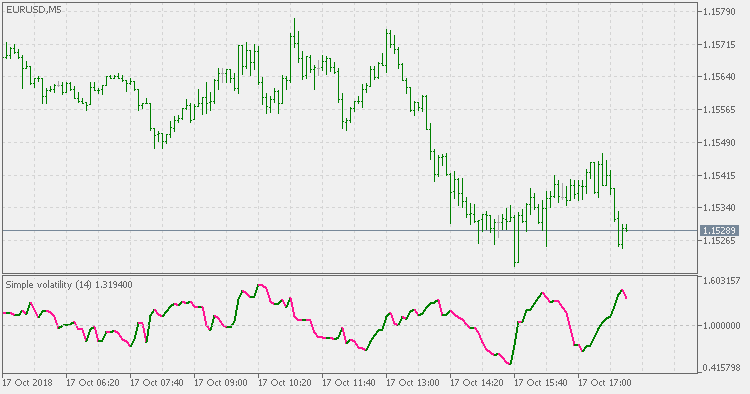Interesting script?
So post a link to it -
let others appraise it

You liked the script? Try it in the MetaTrader 5 terminal# Simple volatility - indicator for MetaTrader 5

Views:
1642
Rating:
Published:
2018.10.17 17:05

In a lot of cases we need some way to measure the volatility

Among the other ways, here is one simple way : it is a ratio of (sum of price differences) to average of (sum of price differences). As simple as it gets, it seems to be detecting the market volatility in acceptable way. As a point of reference :

• values above 1 are periods of increased volatility
• values bellow 1 are periods of decreased volatility

As it can be seen it detects the volatility change OK even in a "thin" market (when the average difference is small and when even a small change is and should be treated as significant volatility change)Ehlers Fisher transform

Ehleres Fisher transformLeader EMAVolatility adjusted RSIVolatility adjusted WPR